#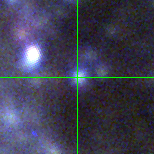M33-013340.60 (J013340.60+304137.1)

## Target Notes### Classification: OB-Supergiant

Unresolved from three LGGS stars of comparable brightness within 2 arcseconds. Also confusion in R and I from red sources in vicinity.

## Plots of Brightness and Color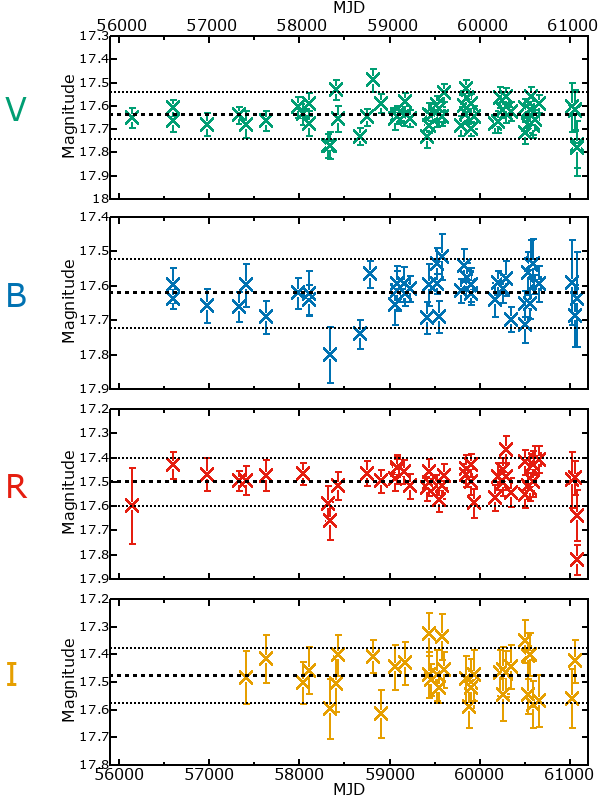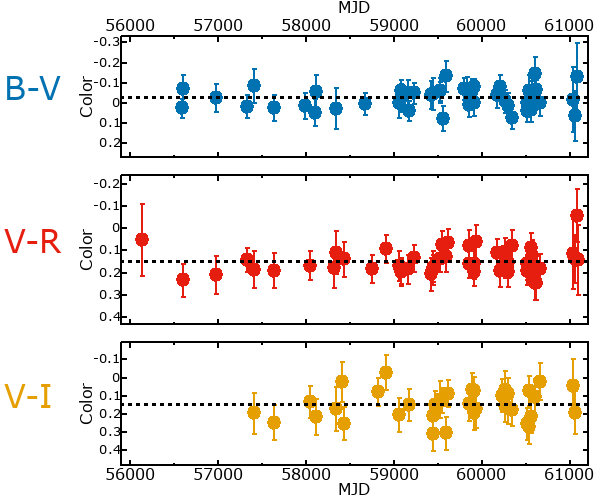## Variability

It is likely that this star is variable.

### Correlation factors between bands

If the brightness variation is true variability then there should be a correlation between brightness changes recorded in different filter bands. The following table are the R-squared linear correlation factors calculated for the pairs of bands. The number in parentheses next to the R-squared value is the number of elements used to calculate the correlation factor. The number in square brackets next to the band is the range of magnitudes measured.

V [0.18 mag]B [0.23 mag]R [0.24 mag]
B [0.18 mag]0.60 (17)
R [0.24 mag]0.60 (17)0.62 (11)
I [0.27 mag]0.04 (11)0.63 (6)0.06 (7)

### Magnitude-Magnitude Plots

The plots below are provided to check the values in the table above and also to show any non-linear correlations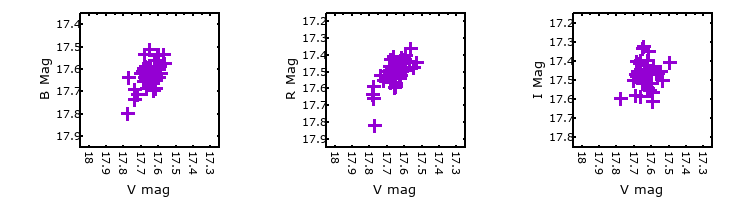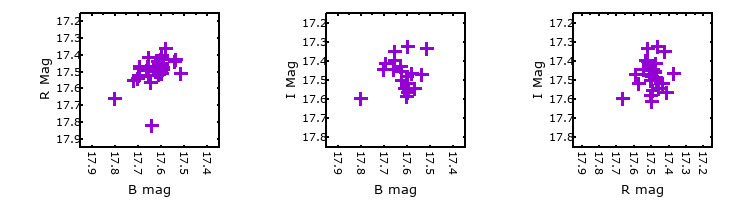### I & R Statistics

Welch & Stetson (1993, AJ, 105, 1813) present a method for varible star detection in CCD images from automated surveys. Data from two bands are compared and two statistics are calculated: R and I.

The I-statistic has an expectation value of zero for stars that are NOT variable. A larger non-zero I-value indicates a higher likelihood that the star is variable. The R statistic indicates how the color of the star changes as the variable grows brighter with R = 1.0 being no change in color.

R and I values have only been calculated if there are more than four (4) matched measurements of brightness in each band.
V
(I|R)
B
(I|R)
R
(I|R)
B
(I|R)
0.73 | 1.09
R
(I|R)
0.57 | 1.120.65 | 0.23
I
(I|R)
0.20 | 1.900.38 | 1.960.07 | 0.93